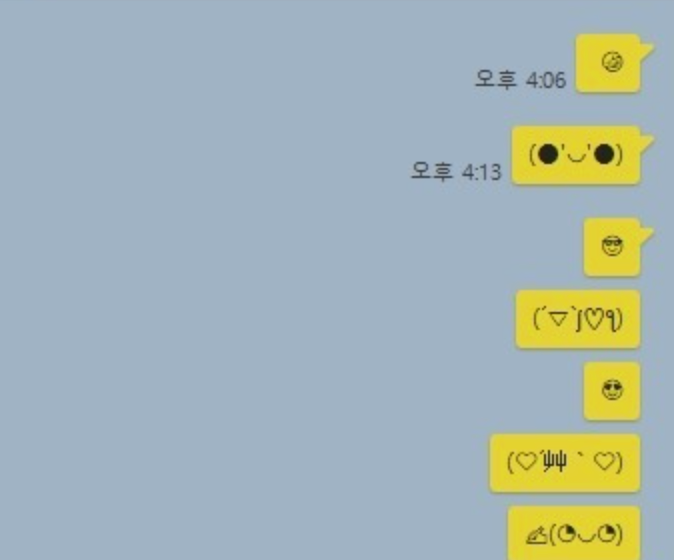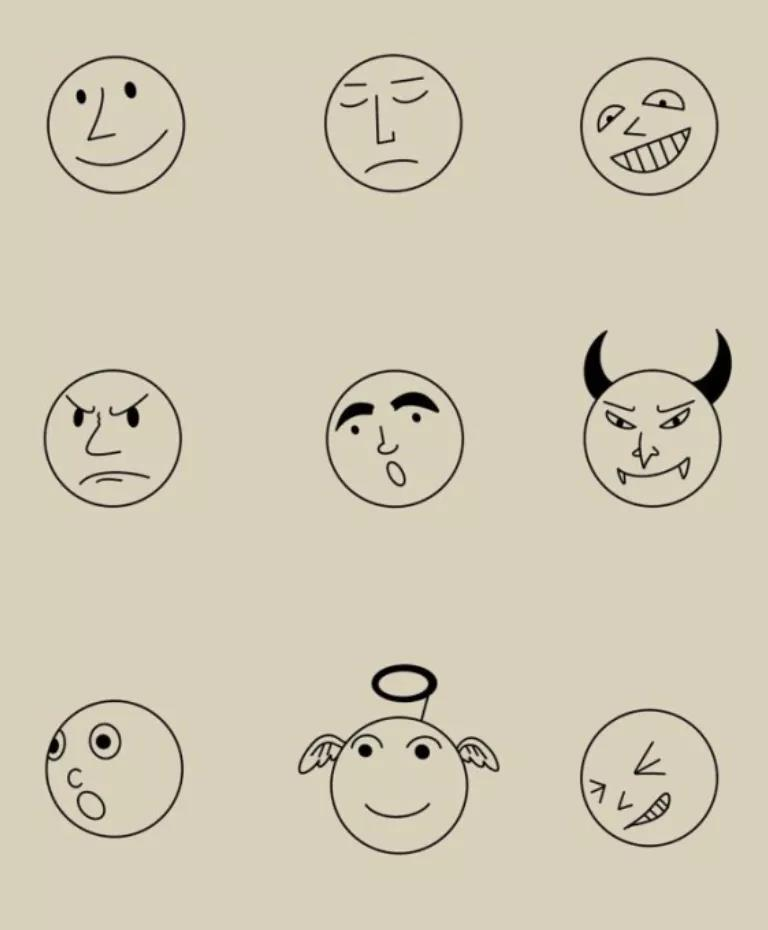• 官方微信• 客服微信### 去韩国留学交流是不是经常无法理解的符号或表1）韩语笑脸表情符号

^^ 笑眯眯的眼睛流露出幸福
^o^ 笑脸（圆嘴）
^_^ 笑脸（直嘴）
^.^ 笑脸（嘴巴可爱）
^-^ 笑脸（小嘴）
^ㅂ^ 笑脸（张开嘴）
n_n 笑脸（大眼睛）
'ㅂ' 笑脸（张开嘴）
(^o^) 笑脸和嘴巴，带有脸部轮廓
*(^o^)* 非常开心的笑脸
★^^★ 笑脸
^_________^ 灿烂的笑容

★.★ 兴奋
(^_^)/ 举起你的手挥手说“嗨”
*_* 激动
d(^_^)b 竖起大拇指
ㅇㅅㅇ 动物鼻子笑脸
•ㅅ• 动物鼻子笑脸（变体）
(•¯ ∀ ¯•) 可爱的动物脸

### 2）韩语悲伤表情符号

ㅜㅜ 含着泪水的悲伤的眼睛
ㅜ.ㅜ 哭脸（小嘴）
ㅜ_ㅜ 哭脸（直嘴）
ㅠㅠ 悲伤的双眸
ㅠ.ㅠ 哭脸（小嘴）
ㅠ_ㅠ 哭脸（直嘴）
T.T 泪眼含泪
Y.Y 悲伤的眼睛里含着泪水
;_; 眼泪汪汪的小眼睛
!_! 眼泪汪汪的大眼睛
OTL 在痛苦或悲伤中蹲在地上（代表四肢着地的人）

### 3）调皮韩语表情

^.~ 眨眼
^3^ 皱起的嘴唇
♡.♡ 爱的眼睛
*^.^* 脸红（也许是赞美）
(>^_^)> <(^_^<) 拥抱（两个人互相拥抱）

### 4）其他韩语表情

ㅇ_ㅇ 惊讶
O_O 震惊了
^^; 慌乱的；尴尬的
^_^;; 因尴尬或尴尬而出汗
=.= 因言语或无聊而心烦意乱或不知所措
-_- 心烦意乱、不知所措或无聊
=_= 因言语而心烦意乱、不知所措或无聊
(-.-)Zzz 睡觉
@_@ 困惑（也可能意味着头晕）
ㅇ_O 不相信
*O* 震惊了
o_o 困惑的笑脸
//_// 害羞的脸
ㅅ'ㅗ 给某人“手指”
V(^-^)V 通过扔“胜利”标志摆姿势
요TL 四肢着地呕吐

치킨 먹자~ （我们吃鸡肉吧~）

안녕~ （你好）

보고싶어~^^（我想你~^^）

1） ㅋㅋㅋ

2） ㅎㅎㅎ

3）헤헤헤024-22523845